# Generate Sine Wave

This example shows how to use the NCO block to generate a sine wave.

The example generates a sine wave with these specifications.

F0 = 510; % Desired output frequency (Hz) Deltaf = 0.05; % Frequency resolution (Hz) SFDR = 90; % Spurious free dynamic range (dB) Ts = 1/8000; % Sample period (s) phOffd = pi/2; % Desired phase offset (rad) 

The frequency resolution of the sine wave depends on the size of the accumulator. Calculate the number of required accumulator bits to achieve the desired frequency resolution. N must be an integer value. Use this value to set the accumulator data type Word length parameter.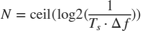N = ceil(log2(1/(Ts*Deltaf))) 
N = 18 

Quantizing the output of the accumulator allows you to achieve better frequency resolution without increasing the lookup table size. Calculate the number of quantized accumulator bits from the spurious free dynamic range specification. Ensure that the Quantize phase parameter is selected, and then use this value to set the set the Number of quantizer accumulator bits parameter.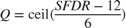Q = ceil((SFDR - 12)/6) 
Q = 13 

Calculate the phase increment. Use this value to set the Phase increment parameter.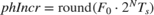phIncr = round(F0*2^N*Ts) 
phIncr = 16712 

Phase offset and dither are optionally added in the accumulator stage. Calculate the phase offset that the block adds from the desired phase offset of the output wave. Use this value to set the Phase offset parameter.phOff = (2^N*phOffd)/(2*pi) 
phOff = 65536 

Select the number of dither bits. In general, a good choice for the number of dither bits is the accumulator word length minus the number of quantized accumulator bits. Use this value to set the Number of dither bits parameter.

ditherBits = N - Q 
ditherBits = 5 

Open and simulate the model. The NCO block in the model is configured with the variables calculated in this script. The output word length and fraction length depend on the constraints of your hardware; this example uses a word length of 16 and a fraction length of 14.

open_system('GenerateSineWaveHDL') sim('GenerateSineWaveHDL')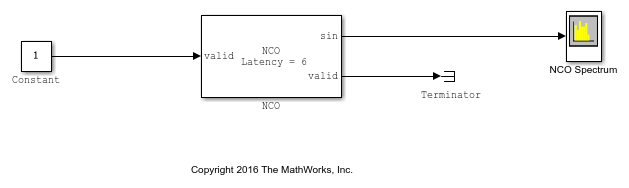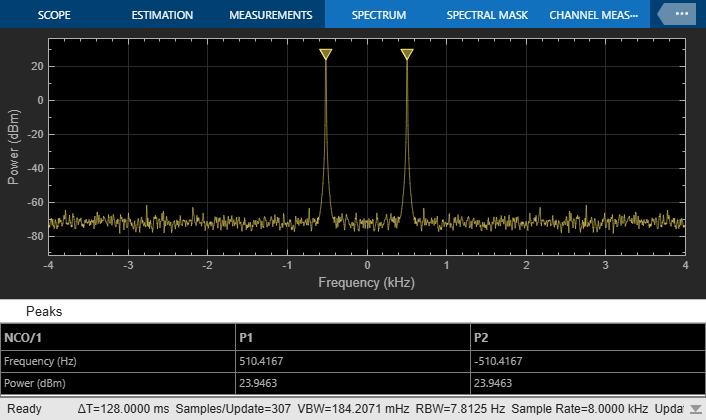The Peak Finder results in the Spectrum Analyzer show that the output waveform is generated at 510 Hz. Experiment with the model to observe the effects on the output shown on the Spectrum Analyzer. For example, try turning dithering on and off, and try changing the number of dither bits.